## Divide a Proper Fraction by a Proper Fraction

Practice Unlimited Questions

#### How to divide a proper fraction by a proper fraction?

Dividing a fraction by another fraction is the same as multiplying the first fraction by the inverse of the second fraction. Here are the steps:

Step 1:   Change  ÷  to  × .
Step 2:   Invert the second fraction.
Step 3:   Simplify the expression.

Example:
Divide
 4 9
by
 2 3
.
 4 9
÷
 2 3
=
 4 9
×
 3 2
=
 2 3

#### 1. If a pizza is cut into quarters, how many slices do you get?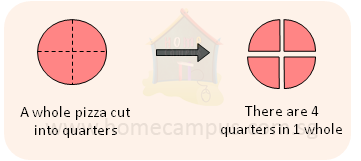1  ÷
 1 4
=  4

You get 4 slices.

#### 2. If you have half a pizza and you cut that into quarters, how many slices do you get?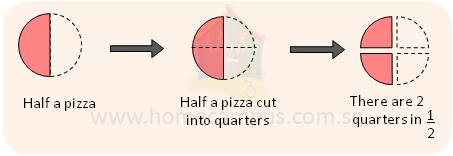1 2
÷
 1 4
=  2

You get 2 slices.

#### 3. If you have    2 3 of a pizza that you cut into one-sixths, how many slices do you get?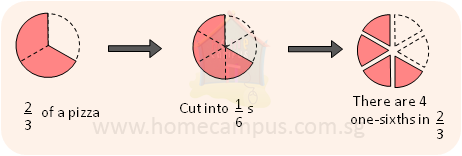2 3
÷
 1 6
=  4

You get 4 slices.

#### 4. Ray has     5 6 litre of tea in a kettle. He pours     1 12 litre of tea into each of some teacups. How many teacups does he fill?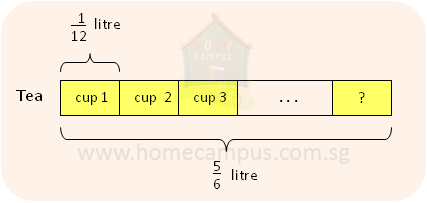5 6
÷
 1 12

 5 6
×
 12 1

=  10

She fills 10 teacups.

#### 5. Maya had     3 4 kg of rice. She packed the rice into bags of     1 10 kg each. a)   How many bags did she make? b)   How much rice was left unpacked? c)   How much more rice does she need to make another bag of     1 10 kg?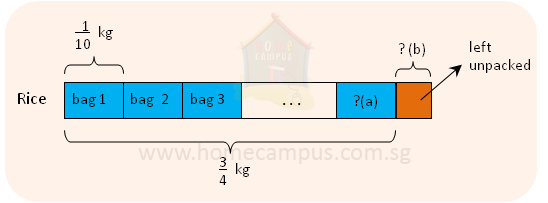a)
 3 4
÷
 1 10

=
 3 4
×
 10 1

=
 30 4

=
 6 1 2

b)     6  ×
 1 10
=
 3 5

There was
 3 5
kg of rice in 6 bags altogether.

 3 4
−
 3 5
=
 1 20

There was
 1 20
kg rice that was left unpacked.

c)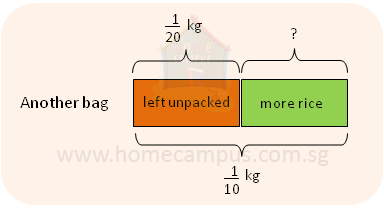1 10
−
 1 20
=
 1 20

She needs
 1 20
kg more rice to make another bag of
 1 10
kg.

#### 6. A rectangular piece of land     1 3 km long has an area of     1 12 km2. Find the length of fence needed to border the land.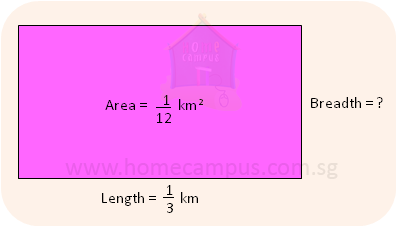Area of the rectangular land  =  length  ×  breadth

 1 12
km2  =
 1 3

 1 12
÷
 1 3

 1 12
×
 3 1

 1 4
km

Border or Perimeter
=  (2  ×  length)  +  (2  ×  breadth)
=  (2  ×
 1 3
)  +  (2  ×
 1 4
)
 2 3
+
 1 2

 7 6
km
 1 1 6
km

The length of fence needed to border the land is
 1 1 6
km.